Latest Banking jobs   »

# Reasoning Ability Quiz For SIDBI GRADE A 2023- 13th January

Directions (1-5): Study the following information carefully and answer the given questions.
Eight students have seated around a circular table in which some are facing towards the centre and some are facing outside the centre. E sits third to the right of D. Only two persons sit between G and B and both are facing same direction. A sits third to the right of the one who sits second to the left of G. F is neither immediate neighbour of G nor B. C sits third to the right of F who sits opposite to H. G is facing same direction as D but opposite to C and A. E sits second to the right of H who is facing same direction as F. B and D faces each other. E faces towards the center.

Q1. Who among the following sits third to the right of A?
(a) E
(b) F
(c) D
(d) G
(e) None of these

Q2. Who among the following sits opposite to C?
(a) B
(b) E
(c) H
(d) A
(e) None of these

Q3. How many persons sits between D and H when counting left of D?
(a) Four
(b) Three
(c) Two
(d) One
(e) None

Q4. What is the position of B with respect to F?
(a) Third to the left
(b) Immediate right
(c) Second to the right
(d) Immediate Left
(e) None of these

Q5. How many persons facing inside?
(a) One
(b) Four
(c) Three
(d) Two
(e) None of these

Direction (6-10): In the question below three statements are given followed by the conclusions. You have to take the given statements to be true even if they seem to be at variance with commonly known facts. Read all the conclusions and then decide which of the given conclusions logically follows from the given statements disregarding commonly known facts.

Q6. Statements: No Quilts are Woolen.
Only a few Woolen are Fabrics.
Only a few Fabrics are Silk.
Conclusions: I. Some Quilts are not Fabrics
II. Some Silk are not Woolen
(a) If only conclusion I follows.
(b) If only conclusion II follows.
(c) If either conclusion I or II follows.
(d) If neither conclusion I nor II follows.
(e) If both conclusions I and II follow.

Q7. Statements: Only a few Shades are Camps.
Some Camps are Fire.
All Fires are Music.
Conclusions: I. All Camps are Fire
II. Some Camps are not Fire.
(a) If only conclusion I follows.
(b) If only conclusion II follows.
(c) If either conclusion I or II follows.
(d) If neither conclusion I nor II follows.
(e) If both conclusions I and II follow.

Q8. Statements: Only a few Ears are Earrings.
No Earrings are Fiber.
Only a few Fiber are Goggles.
Conclusions: I. Some Ears are not Fiber
II. Some Goggles are not Earrings.
(a) If only conclusion I follows.
(b) If only conclusion II follows.
(c) If either conclusion I or II follows.
(d) If neither conclusion I nor II follows.
(e) If both conclusions I and II follow.

Q9. Statements: Only a few Classic are Western.
Only a few Western are Jazz.
All Western and Jazz are Salsa.
Conclusions: I. Some Jazz are not Classic
II. All Classic can be Salsa.
(a) If only conclusion I follows.
(b) If only conclusion II follows.
(c) If either conclusion I or II follows.
(d) If neither conclusion I nor II follows.
(e) If both conclusions I and II follow.

Q10. Statements: Only a few Rhymes are Poems.
Some Poems are not Limericks.
Some Limericks are not Verses.
Conclusions: I. Some Rhymes are Limericks.
II. No Rhymes are Limericks.
(a) If only conclusion I follows.
(b) If only conclusion II follows.
(c) If either conclusion I or II follows.
(d) If neither conclusion I nor II follows.
(e) If both conclusions I and II follow.

Directions (11-15): In these questions, relationship between different elements is show in the statements. The statements are followed by conclusions. Study the conclusions based on the given statements and select the appropriate answer:

Q11.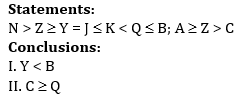(a) Both conclusions I and II are true
(b) Either conclusion I or II is true
(c) Only conclusion I is true
(d) Neither conclusions I nor II is true
(e) Only conclusion II is true

Q12.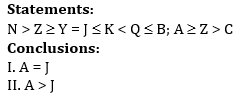(a) Both conclusions I and II are true
(b) Only conclusion II is true
(c) Neither conclusion I nor II is true
(d) Either conclusions I or II is true
(e) Only conclusions I is true

Q13.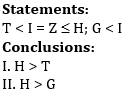(a) Both conclusion I and II are true
(b) Either conclusion I or II is true
(c) Neither conclusion I nor II is true
(d) Only conclusion I is true
(e) Only conclusion II is true

Q14.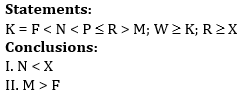(a) Only conclusion II is true
(b) Either conclusion I or II is true
(c) Both conclusion I and II are true
(d) Neither conclusion I nor II is true
(e) Only conclusion I is true

Q15.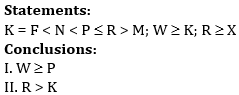(a) Neither conclusion I nor II is true
(b) Both conclusions I and II are true
(c) Only conclusion II is true
(d) Either conclusion I or II is true
(e) Only conclusion I is true

Solutions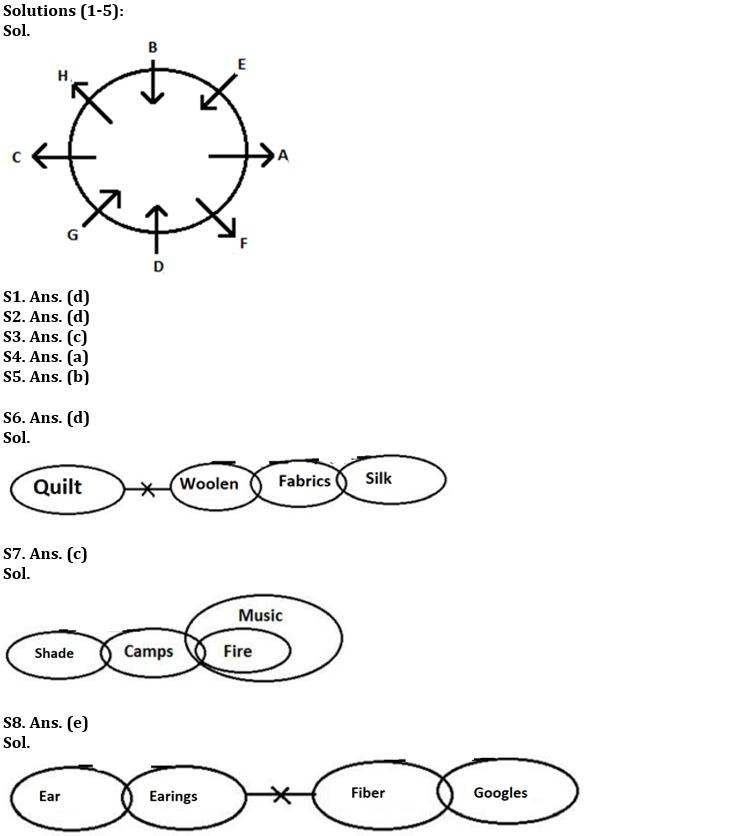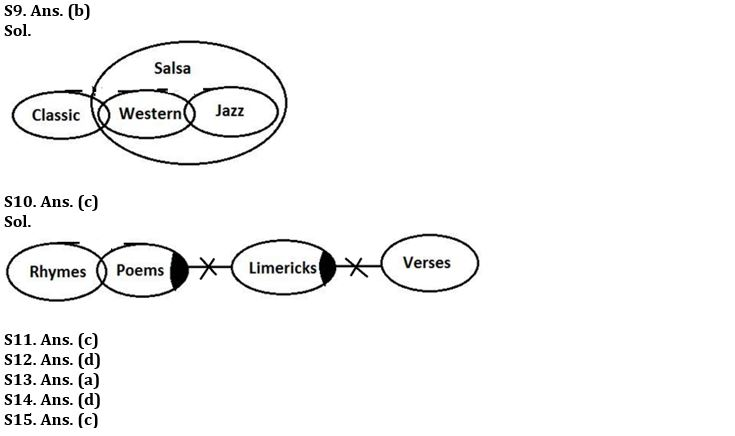## FAQs

### When will the SIDBI Grade A Exam 2023 be held?

The SIDBI Grade A Exam 2023 will be held on 28th January 2023.

#### Congratulations!